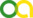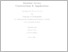(2021). Modular Forms: Constructions & Applications. PhD thesis, Universität zu Köln.Preview
PDF
ThesisMain.pdf - Accepted Version
Bereitstellung unter der CC-Lizenz: Creative Commons Attribution.

## Abstract

This thesis combines results of five research papers on the construction and applications of modular forms and their generalisations. We begin by constructing new examples of quantum modular forms of depth two, generalising results of Bringmann, Kaszian, and Milas. To do so, we relate the asymptotics of certain false theta functions of binary quadratic forms to multiple Eichler integrals of theta functions. Quantum modularity of the false theta functions follows from the behaviour of such integrals near the real line. Next, we turn our attention to the asymptotic profile of a certain eta-theta quotient that arises in the partition function of entanglement entropy in string theory. In particular, we generalise methods of Bringmann and Dousse, and Dousse and Mertens, to deal with the meromorphic Jacobi form at hand. Applying Wright's circle method for Jacobi forms we obtain a bivariate asymptotic for the two-variable coefficients of the eta-theta quotient. Thirdly, we investigate the asymptotic behaviour of the generating function of integer partitions whose ranks are congruent to r modulo t, denoted by N(r; t; n). By proving that the series has monotonic increasing coefficients above some bound, we are in a position to apply Ingham's Tauberian theorem. This immediately implies that N(r; t; n) is equidistributed in r for fixed t as n tends to infinity, in turn implying a recent conjecture of Hou and Jagadeeson on a convexity-type result. The following chapter is dedicated to an investigation of traces of cycle integrals of meromorphic modular forms and their relationship to coefficients of harmonic Maass forms. Working on lattices of signature (1,2), we first relate a locally harmonic Maass form to a Siegel theta lift involving the Maass raising operator by explicitly computing the raising of the locally harmonic Maass form, and using the usual unfolding argument for the theta lift. We then borrow techniques of Bruinier, Ehlen, and Yang to compute the theta lift as (up to terms that vanish for certain classes of input functions) the constant term in a q-series involving the coefficients of xi-preimages of unary theta functions as well as theta functions. Since such preimages are harmonic Maass forms, we obtain a description of the traces in terms of coefficients of theta functions and harmonic Maass forms. Choosing a specific lattice related to quadratic forms and noting that the functions determining the constant term can be chosen to have rational coefficients, we obtain a new proof of a recent result of Alfes-Neumann, Bringmann, and Schwagenscheidt. Finally, we investigate the relationship between modular forms and self-conjugate t-core partitions. We obtain the number of self-conjugate 7-cores as a single class number in two ways. The first we show with modularity arguments on the generating function of Hurwitz class numbers. We also provide a complementary combinatorial description to explain the equality. In particular, we construct an explicit map between self-conjugate t-cores and quadratic forms in a given class group. Moreover, we show that the genus of the quadratic forms is unique, and determine the number of preimages of the genus. Using these results, we show an equality between the number of 4-cores and the number of self-conjugate 7-cores on specific arithmetic progressions. Aside from the t = 4 case, we consider whether equalities between t-cores and self-conjugate 2t-1-cores are possible. We show for t = 2,3,5 that they are not, and offer a conjecture and partial results for t > 5.

Item Type: Thesis (PhD thesis)
Translated abstract:
AbstractLanguage
UNSPECIFIEDGerman
Creators:
CreatorsEmailORCIDORCID Put Code
Males, Joshuajmales@math.uni-koeln.deorcid.org/0000-0002-0324-601XUNSPECIFIED
URN: urn:nbn:de:hbz:38-467368
Date: 6 May 2021
Language: English
Faculty: Faculty of Mathematics and Natural Sciences
Divisions: Faculty of Mathematics and Natural Sciences > Department of Mathematics and Computer Science > Mathematical Institute
Subjects: Mathematics
Uncontrolled Keywords:
KeywordsLanguage
Modular formsEnglish
Harmonic Maass formsEnglish
Asymptotics and the circle methodEnglish
Date of oral exam: 5 May 2021
Referee:
Bringmann, KathrinProf. Dr.
Zwegers, SanderProf. Dr.
Refereed: Yes
URI: http://kups.ub.uni-koeln.de/id/eprint/46736View Item